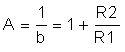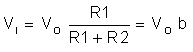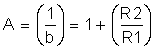SLOA011B January   2018  – July 2021

1. 1Introduction
2. 2Non-Inverting Amplifier
3. 3Inverting Amplifier
4. 4Simplified Op Amp Circuit Diagram
5. 5Op Amp Specifications
6. 6References
7. 7Glossary
8. 8Revision History

## 2.1 Closed Loop Concepts and Simplifications

Substituting a = ∞ Equation 1 into Equation 16 results in,

Equation 17.Recall that in equation Equation 6 we state that Vd, the voltage difference between Vn and Vp, is equal to zero and therefore, Vn = Vp. Still they are not shorted together. Rather there is said to be a virtual short between Vn and Vp. The concept of the virtual short further simplifies analysis of the non-inverting op amp circuit in Figure 2-1.

Using the virtual short concept, we can say that,

Equation 18. Vn= Vp = Vi

Realizing that finding Vn is now the same resistor divider problem solved in Equation 12 and substituting Equation 18 into it, we get,

Equation 19.Rearranging and solving for A, we get,

Equation 20.The same result is derived in Equation 17. Using the virtual short concept reduced solving the non-inverting amplifier, shown in Figure 2-1, to solving a resistor divider network.